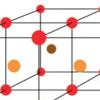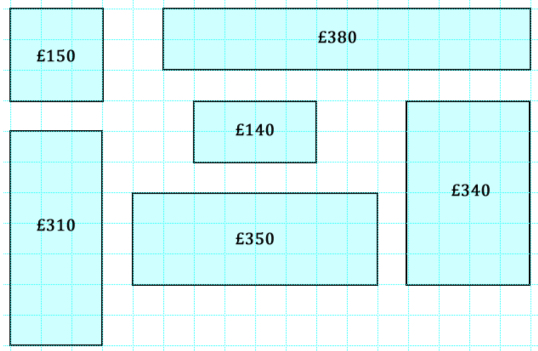#### You may also likeThink of a number and follow the machine's instructions... I know what your number is! Can you explain how I know?### Diagonal Sums

In this 100 square, look at the green square which contains the numbers 2, 3, 12 and 13. What is the sum of the numbers that are diagonally opposite each other? What do you notice?### Finding 3D Stacks

Can you find a way of counting the spheres in these arrangements?

# Price Match

##### Age 7 to 11Challenge Level

You might like to try Through the Window before this challenge.

In a particular shop, the price of windows is calculated according to the area of glass used and the length of frame needed.

In the picture below, glass costs  £10 for an area of one small square, plus £5 for every square edge of frame.If we call the area of the glass A and the perimeter of the window P, how would you express the cost of the window in terms of A and P?

Use your formula to find pairs of rectangular windows that are different sizes but cost the same.